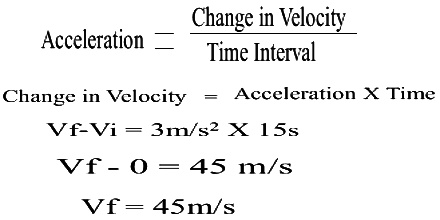# Acceleration Related to Motion

Acceleration: Whenever there is a change of velocity of a body, we say that the body is accelerated. The quantity which is used to express the successive change of velocity of a body or the rate of change of velocity of a body with time is called acceleration.

Generally, rate of change of velocity with time is called acceleration. Time rate of change of velocity of a body is called acceleration. This means that the velocity is changing, so from the definition of variable velocity we can say that variable velocity is also called acceleration.You are accelerating when you change speed or direction.

• You change speed when you slow down. So if you slow down at a red light, you are accelerating (often called deceleration or negative acceleration).
• You change speed when you go faster. When you are merging onto a freeway and speed up to fit into the flow of traffic you are accelerating.
• You accelerate when you turn a corner or go around a curve even if you speed remains the same.

Example

An airplane accelerates down a runway at 3.20 m/s2 for 32.8 s until is finally lifts off the ground. Determine the distance traveled before takeoff.

Here,

a = + 3.2 m/s2

t = 32.8 s

v1 = 0 m/s

Find: d.

we know, d = v1t + 0.5 at2

d = (0 m/s) (32.8 s)+ 0.5 (3.20 m/s2)*(32.8 s)2

so, d = 1720 m